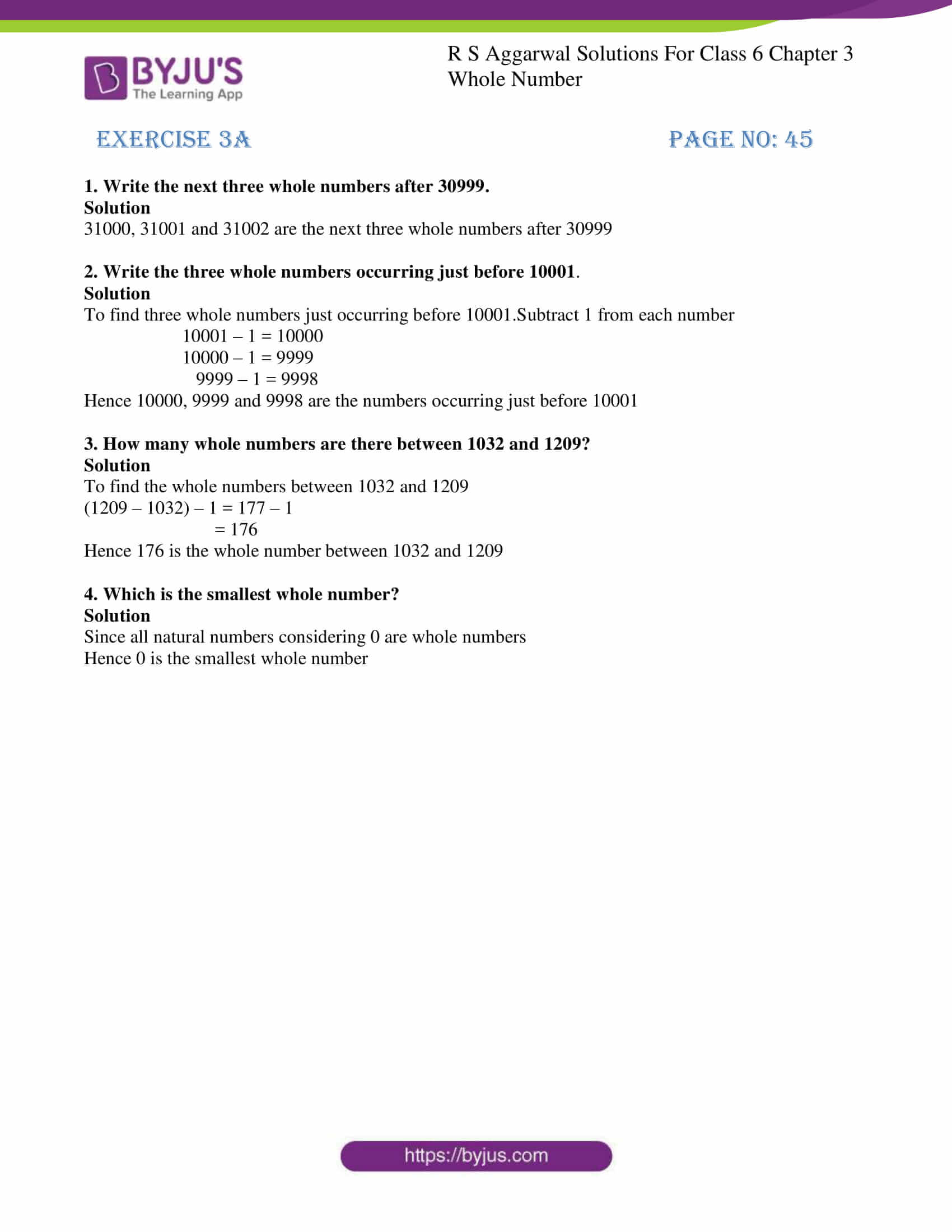# RS Aggarwal Solutions for Class 6 Chapter 3 Whole Numbers Exercise 3A

RS Aggarwal Solutions for Class 6, Chapter 3, Whole Numbers Exercise 3A in simple PDF are given here. This exercise contains questions related to the successor and predecessor of whole numbers. The successor of the whole number is the addition of 1 to a whole number, whereas the predecessor of a whole number is the subtraction of 1 from a whole number. Learn more about these topics by solving the questions using RS Aggarwal Solutions for Class 6 Chapter 3 Whole Numbers Exercise 3A provided here.

## Download PDF of RS Aggarwal Solutions for Class 6 Chapter 3 Whole Numbers Exercise 3A### Access answers to Maths RS Aggarwal Solutions for Class 6 Chapter 3 Whole Numbers Exercise 3A

1. Write the next three whole numbers after 30999.

Solution

31000, 31001 and 31002 are the next three whole numbers after 30999

2. Write the three whole numbers occurring just before 10001.

Solution

To find three whole numbers just occurring before 10001.Subtract 1 from each number

10001 – 1 = 10000

10000 – 1 = 9999

9999 – 1 = 9998

Hence 10000, 9999 and 9998 are the numbers occurring just before 10001

3. How many whole numbers are there between 1032 and 1209?

Solution

To find the whole numbers between 1032 and 1209

(1209 – 1032) – 1 = 177 – 1

= 176

Hence 176 is the whole number between 1032 and 1209

4. Which is the smallest whole number?

Solution

Since all natural numbers considering 0 are whole numbers

Hence 0 is the smallest whole number